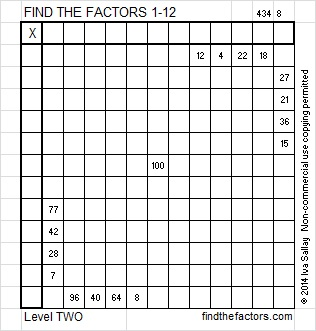# 214 and Level 2

• 214 is a composite number.
• Prime factorization: 214 = 2 x 107
• The exponents in the prime factorization are 1 and 1. Adding one to each and multiplying we get (1 + 1) x (1 + 1) = 2 x 2 = 4. Therefore 214 has 4 factors.
• Factors of 214: 1, 2, 107, 214
• Factor pairs: 214 = 1 x 214 or 2 x 107
• Since 214 has no square factors, the square root of 214 cannot be simplified. √214 ≈ 14.629Excel file of puzzles and previous week’s factor solutions: 12 Factors 2014-08-25